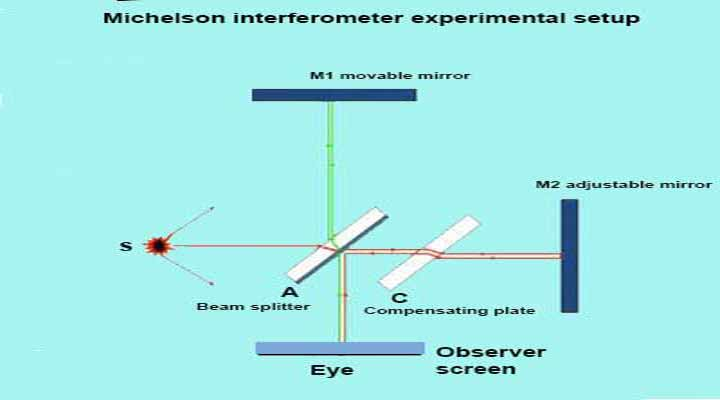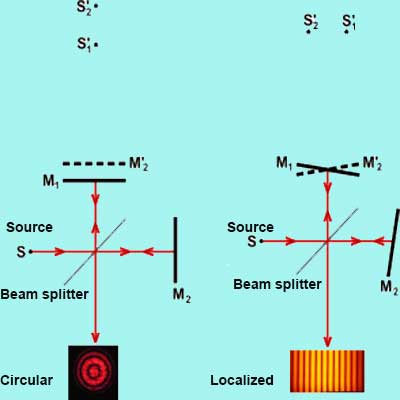# Michelson Interferometer, Definition, Diagram, Derivation, Setup, images, applications

0
12634Michelson Interferometer

Albert Abraham Michelson: Which was an American physicist who developed an instrument using the concept of interferometry, the so-called Michelson interferometer.

It is used to determine the wavelength of light and the refractive index of thin material. “Michelson interferometer diagram and derivation” Let us start.

In this experiment, a monochromatic source of light is used to produce fringes of light by splitting a beam of light into two arms by the use of a beam splitter.

These light beams reflected back towards the beam splitter, which then combines their amplitude using the superposition principle and interference pattern results.## Michelson Interferometer Working.

In this experiment, two polished mirrors are used (let M1 and M2). These mirrors are perfectly perpendicular to each other.

Two glass plates A and C are placed between the two mirrors.

The backside of glass plate A is half polished so, half of the light is reflected towards mirror M1 and half transmitted towards mirror M2.

After that, these two beams of light reflected back by the M1 and M2 mirrors to the plates.

The wave reflected from the mirror M1 is transmitted through the plate A and the wave reflected from M2 is reflected again by the glass plate A

The two waves from mirror M1 and M2 interfere and fringes are observed by the observer.

Also, constructive and destructive interference occurs depending on the relative phase shift between two plane waves.

### Michelson Interferometer compensating plate.

This experimental setup produces an extra optical path like the wave which reflected from the mirror M1 and passes through plate A twice.

Another plate is placed in the path of the reflected wave from the mirror M2 so that the wave passes through this is also twice.

So, to create balance in these two waves this (C named ) plate is used. This is called a compensating plate.

### Michelson Interferometer condition for constructive interference.

The path difference between the two waves must be an integral multiple of .

Where m is the order and m= 0,1,2,3,….. and λ is the wavelength. In constructive interference the fringes are bright.

Δ=2d cosθ+λ /2 = ( total path difference between the two waves)

Δ=2d cosθ+λ /2 = mλ, m=0, 1, 2,… For constructive interference

### Michelson Interferometer condition for destructive interference.

If the path difference between the two waves is (m+½)λ.

Then the fringes appear is dark.

Δ=2d cosθ+λ /2 = (m+½)λ,  m=0, 1, 2,… For destructive interference

In this experiment, many fringes forms are illustrated below.

1 Circles fringes.

2 Straight fringes.

3 Ellipses fringes.

4 Hyperbolae fringes.

These fringes formation depends upon the distance d between M1 and M2′ and the angle between these surfaces.

Here M2′ is the virtual image of M2 formed in glass plate A for the observer, who sees in the direction of M1.## Michelson Interferometer fringes

### Circular fringes

Circular fringes are forms when the two mirrors are perfectly in a perpendicular direction to each other and M1 is parallel to M2′ and the angle is zero.

Let the distance between M1 and M2′ is equal to d.

The if the distance between virtual images S1′ and S2′ of source S due to parallel M1 and M2′ is equal to 2d.

The virtual sources in S1′ and S2′ are said to be in phase to each other (Such sources are called coherent sources) and in that the phases of corresponding points in the are exactly the same at all times.

Now the path difference between the two incoming waves will be equal to 2d cosθ.

where the θ is the angle between the viewing axis and the incoming falling wave.

Here the characteristics of the interference pattern depend on the nature of the light source and the precise orientation of the mirror and beam splitter.

Now the parallel waves interfere with each other and produced a fringe pattern of maxima and minima for which 2d cosθ = mλ.

Here the optical elements are oriented so that S1′ and S2′ are in line with the observer and pattern in resulting circular forms on the normal M1 and M2′.

These are called fringes of equal inclination

### Localized fringes (straight or parallel)

In which M1 and M2′ are overlapped and also not observed for large path difference (fringes with equal thickness).

#### Michelson Interferometer Wavelength.

We know that in constructive interference bright fringes visible in the field of view.

So if we adjust the position of mirror M1 in such a way, for which it satisfies the condition of constructive interference, increasing d by λ ⁄2 and path difference between the reflected waves becomes 2(d+λ ⁄2). Here we assumed cosθ=1

Hence, at last, the wavelength will be d=mλ ⁄ 2 or λ=2d ⁄m ( n=order of fringes).

#### Michelson Interferometer experimental precautions.

1 Always handles the screw with gently because it can break the mirror.

2 Never touch the lens or mirrors with your fingers, when you working with them.

3 Always in touch with your instructor for further information. While you working with a laser, as a source of light always be careful.

Never look at the laser beam directly as most of the laser beams have high intensity and it can damage your eye’s retina.

## Application of Michelson Interferometer.

1 It is used in astronomical interferometry and also used in coherence tomography.

2 It used in the analysis of the upper atmosphere, by indicating the winds and temperature.

This instrument is used to measuring the Doppler width and shifting in the spectrum of airglow and also Aurora.

3 Many operations are done with the Michelson interferometer of Fourier transform spectrometer for adjustment of the movable mirror.

The Fourier transform makes changes and converts the interferogram into an actual spectrum.

4 The Fourier transform spectrometers can offer significant advantages over dispersive grating and prism spectrometers under certain conditions.

5 It used in the making of fiber optics materials.

6 To make a tunable narrowband filter.